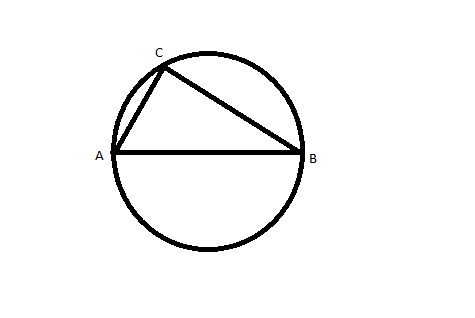Question 19

# The figure shows a circle of diameter AB and radius 6.5 cm. If chord CA is 5 cm long, find the area of triangle ABC.Solution

According to given dimensions, triangle will be a right angled triangle.
So BC = 12
And area = $$\frac{1}{2} \times 12 \times 5$$
= 30

• All Quant CAT Formulas and shortcuts PDF
• 30+ CAT previous papers with solutions PDF